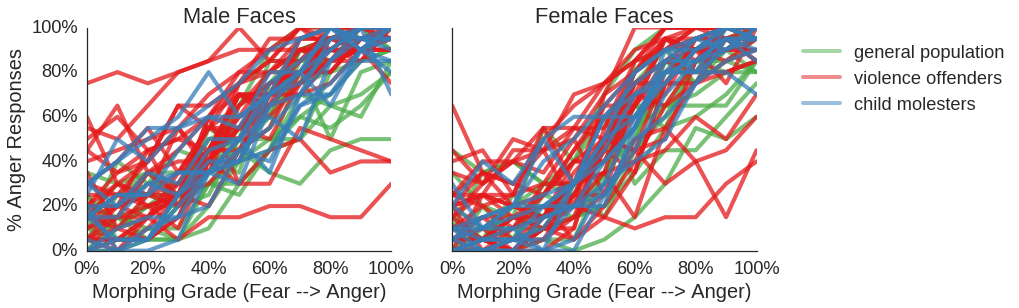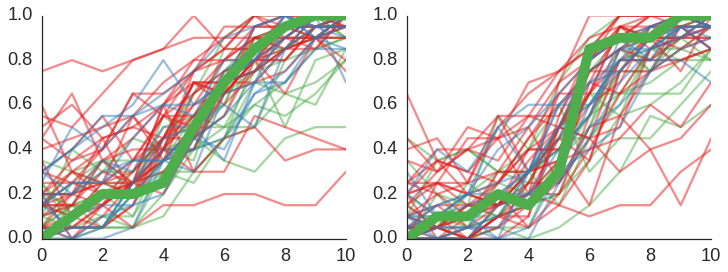# 4. Basic Plotting and Statistics for morphed faces¶

### import libraries¶

In :
import numpy as np
import pandas as pd

import os
import fnmatch

import matplotlib.pyplot as plt
import seaborn as sns
%matplotlib inline

from myBasics import *

In :
bigDf = pd.read_csv('../outputs/genderTable.csv',
index_col=[0,1,2])

In :
bigDf.head()

Out:
00 01 02 03 04 05 06 07 08 09 10 group
g p fgender
A A001 F 0.00 0.10 0.10 0.2 0.15 0.30 0.85 0.90 0.90 1.00 1.00 2
M 0.00 0.10 0.20 0.2 0.25 0.50 0.70 0.85 0.95 1.00 1.00 2
A002 F 0.20 0.10 0.05 0.3 0.25 0.50 0.75 0.90 0.75 1.00 1.00 2
M 0.05 0.25 0.35 0.2 0.35 0.55 0.60 0.90 0.85 1.00 1.00 2
A003 F 0.00 0.05 0.15 0.1 0.30 0.40 0.55 0.65 0.65 0.65 0.85 2

### One plot for each face gender¶

In :
def makeGenderSpaghetti(bigDf,cond,count):

ax = plt.subplot(1,2,count)

# to loop through all cases but have only one legend without redundancies,
# here we keep track of whether a condition is already labeled
legTrack = []

# looping  through the 3 groups
for group in bigDf.index.levels:
# looping through the participants of each group
for p in bigDf.ix[group].index:
if p in group:

# color and legend setting for that group
thisCol = myPal[labelCoding[p]]
thisLeg = myGLabels[p]

# get the data from the specified row
thisList = list(bigDf.ix[group].ix[p].ix[cond])[:-1] # last row is group membership; get rid of that

# plotting with legend if this is the first instance,
# otherwise plot without a legend
if thisLeg not in legTrack:
ax.plot(thisList,
c=thisCol,
alpha=0.5,
linewidth=4,
label=thisLeg)
else:
ax.plot(thisList,
c=thisCol,
linewidth=4,
alpha=0.5)
# keep track which conditions already have a legend
legTrack.append(thisLeg)

# plot formatting

ax.set_ylabel('% Anger Responses')

plt.xticks(np.arange(0,10.1,2), [str(a)+'%' for a in  np.arange(0,101,20)])
plt.yticks(np.arange(0,1.01,0.2), [str(a)+'%' for a in  np.arange(0,101,20)])

#plt.ylim(.0,1.); plt.xlim(-0.1,10.2)
if cond == 'M':
ax.set_title('Male Faces')
elif cond == 'F':
ax.set_title('Female Faces')
ax.set_ylabel('')
ax.set_yticks([])
# one legend for both plots
plt.legend(loc='best',bbox_to_anchor=[1.1, 1])

In :
plt.figure(figsize=(12,4))
for i,fgender in enumerate(['M','F']):
makeGenderSpaghetti(bigDf,fgender,i+1)
sns.despine()
#plt.savefig('../figures/rawSpaghettiAll.png',dpi=300,bbox_inches="tight")
plt.show()Same thing, but as interactive plot

In :
def interactiveSpaghetti(i):

mmDf = bigDf[1::2]
mDf = mmDf[mmDf.columns[:-1]]
mY = list( mmDf['group'] )

ffDf = bigDf[0::2]
fDf = ffDf[ffDf.columns[:-1]]
fY = list( ffDf['group'])

fig = plt.figure(figsize=(12,4))

### male
ax = plt.subplot(1,2,1)

selectDf = mDf.ix[i]
otherDf = np.array( pd.concat([mDf[:i],mDf[i+1:] ] ) )

for entry in range(otherDf.shape):
try: ax.plot(otherDf[entry],alpha=0.5,color=myPal[mY[entry]])
except: print "!"

ax.plot(selectDf,linewidth=10,color=myPal[mY[i]])

### female
ax = plt.subplot(1,2,2)

selectDf = fDf.ix[i]
otherDf = np.array( pd.concat([fDf[:i],fDf[i+1:] ] ) )

for entry in range(otherDf.shape):
try: ax.plot(otherDf[entry],alpha=0.5,color=myPal[fY[entry]])
except: print "!"

ax.plot(selectDf,linewidth=10,color=myPal[fY[i]])
sns.despine()

return fig

In :
# Interactive plots for static html notebooks; using ipywidgets by Jake Vanderplas
# https://github.com/jakevdp/ipywidgets-static

from ipywidgets_static import StaticInteract, RangeWidget, RadioWidget

In :
StaticInteract(interactiveSpaghetti,
i=RangeWidget(0, 60, 1)
)

/opt/anaconda2/lib/python2.7/site-packages/matplotlib/pyplot.py:516: RuntimeWarning: More than 20 figures have been opened. Figures created through the pyplot interface (matplotlib.pyplot.figure) are retained until explicitly closed and may consume too much memory. (To control this warning, see the rcParam figure.max_open_warning).
max_open_warning, RuntimeWarning)

Out: